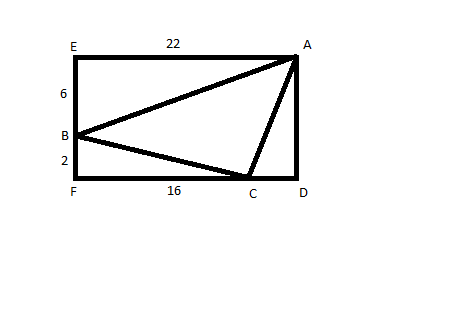Question 73

# In the given figure, EADF is a rectangle and ABC is a triangle whose vertices lie on the sides of EADF and AE = 22, BE = 6, CF = 16 and BF = 2. Find the length of the line joining the mid-points of the sides AB and BC.Solution

$$AC^2 = 8^2 + 6^2 = 100$$
So AC = 10

$$\frac{10}{EF}$$ = 2

EF = 5

Length of line joining mid-points will be = 5

• All Quant CAT Formulas and shortcuts PDF
• 30+ CAT previous papers with solutions PDF

##### deepthiMAHENDAR Maheshuni

3 years, 7 months ago

I know this method

##### Diksha Jaiswal

3 years, 2 months ago

Plz elaborate once

##### Diksha Jaiswal

3 years, 2 months ago

How did 2 come?

OR